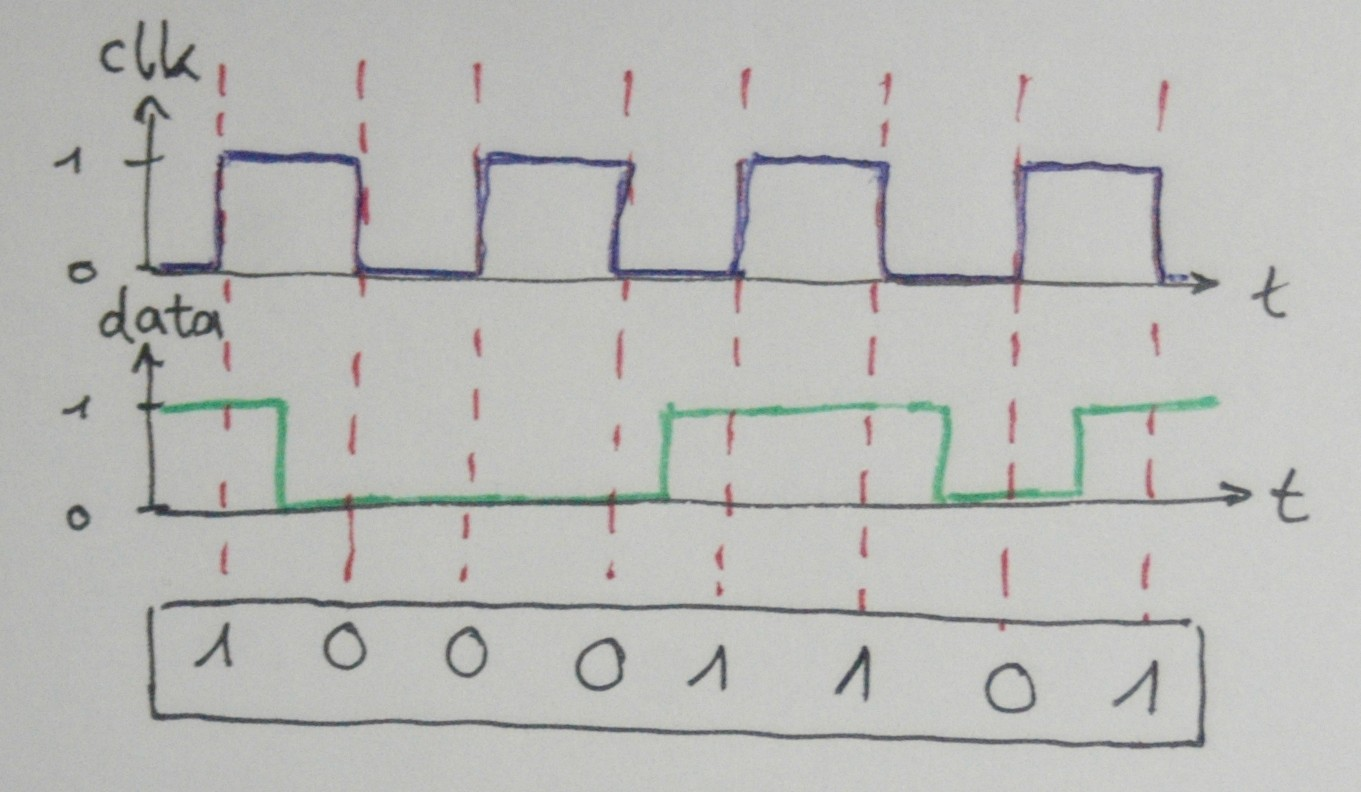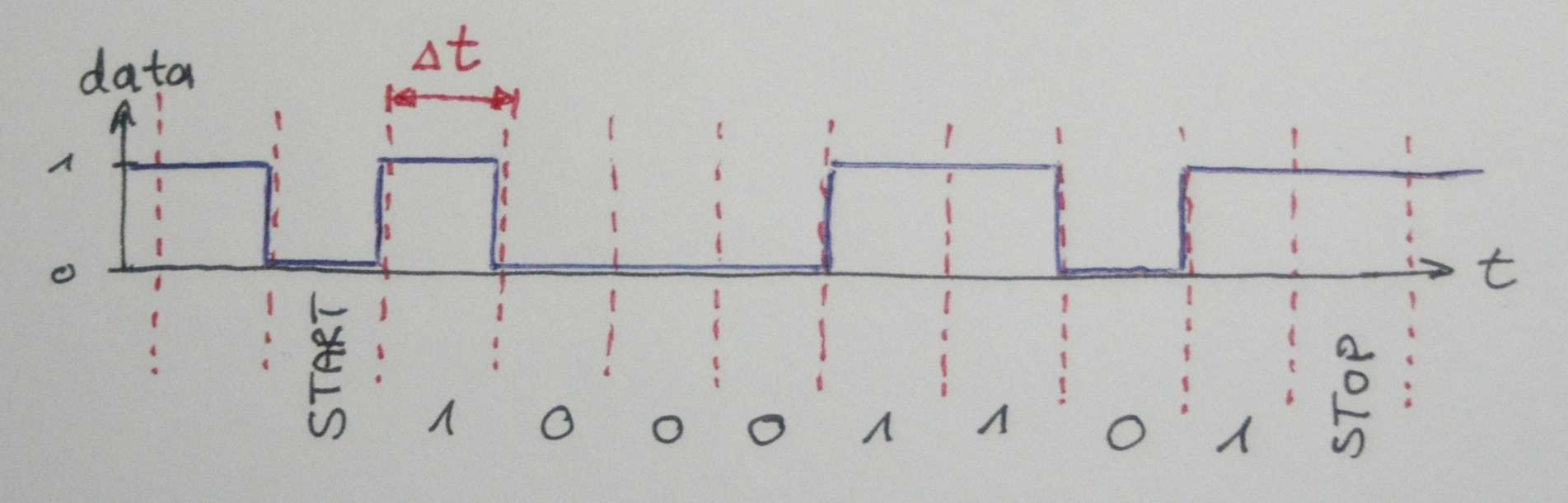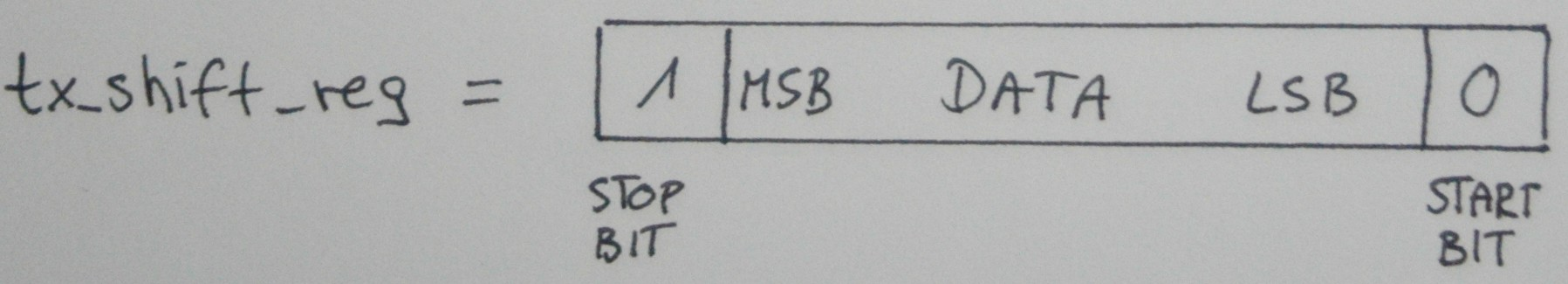a tiny software UART TX for the AVR ATtiny

The serial port is a very commonly used interface for communicating between a microcontroller and a PC for debugging or sending or receiving some values. Most microcontrollers include a hardware peripheral called UART (Universal Asynchronous Receiver Transmitter) which handles the serial communication, but some especially small microcontrollers (like the ATtiny24a that I am using) don’t have one. In this case we can implement the communication in Software.

This approach is often called bit banging and can be used to emulate all sorts of communication peripherals. Another use case might be, that in an existing project the pins tied to the hardware-UART are already in use, in which case we could also use a software-UART. Since it is implemented in software, every GPIO pin can be used.

Now let’s take a quick look at how the serial communication works. As the name says, the communication is asynchronous, but what does that mean?

The opposite is a synchroneous communication protocol, which has a clock signal and one (or more) data signals. At every clock cycle, the receiver knows that there’s a new bit available and reads it. That way the clock frequency controls the rate at which data is being sent. In the below diagram we can see a clock signal (blue) and a data signal (green).In this example at every rising or falling edge the data signal is sampled, i.e. we look at whether it is high (0) or low (1). This way we can receive the data which in this case results to 10001101 in binary or 141 in decimal.

Now in the case of an asynchronous communication protocol, there is no clock signal. Thus, for the receiver to know when to sample the data, he must know two things: when does the data message start and stop, how long is it and at which rate the data is being sent.

To solve the first problem, the serial protocol uses a so called START-bit and a STOP-bit (there are variants that use 2 STOP-bits and/or a parity bit, but these are not very common). When the line is idle, the signal is high (or low, depending of the standard). The first falling edge indicates to the receiver that a message will be sent, the line remains low for the duration of one bit Δt, this is the START-bit.

Afterwards the message bits follow and are terminated by a STOP-bit, i.e. the line remains high for the duration of one bit. In this case, for serial communication the message length is almost always 8 bit. Now thanks to the START-bit the receiver can tell when a message starts, but in order to correctly sample the bits at the right time he must know the duration of one bit.

This means that both receiver and transmitter must use the same rate for sending/receiving, the so called baud rate. In this case, the baud rate corresponds to the bit rate, so if the duration of one bit is Δt, the baud rate is 1/Δt. Some commonly used baud rates are e.g. 9600 (rather slow) or 115200 (faster).

Take a look at the following example diagram:Now what would happen the rate at which the transmitter emits bits and the rate at which the receiver samples them would slightly differ, due to inaccuracies of their system clocks?

If they differ too much, the receiver will sample the signal at the wrong points and the resulting data will be wrong. You can read up further on this in . In practice it means that for a microcontroller to reliably use asynchroneous serial communication, its clock source should derived from an external crystal or ceramic resonator (which are very accurate) and not from the internal RC-oscillator (which is very inaccurate).

Here I will nevertheless use the ATtiny’s internal oscillator, and often you will be lucky and it will work. It it doesn’t, we can tune the clock speed until it matches the one from the PC. But more about that later on.

So now how to implement a simple UART transmitter in software? A very straightforward approach would do something like:

set pin high;
wait(Δt);
set pin low;

and so on to create the desired signal on the output pin. But such a solution has two downsides: first, the delays have to be very accurate as we’ve seen in the previous paragraph. Second, using wait (delays) basically means that our microcontroller just sits around doing nothing and wastes precious computing time until the transmission is finished.

In this approach, we will be using a timer and interrupts, so that in the time between the transmitted bits, the CPU is free to do other calculations. Let’s walk through the code step by step to understand how it works:

First, we use some #defines to set which output pin we will be using. That way we can change the pin quickly.

Then we need a variable that holds our data to be sent.

We will use it like a shift register, therefore the name. Then we need to set up the GPIO pin as an output and set up a timer (here timer 0). We configure the timer in CTC (Clear Timer on Compare Match) mode. This means that the counter will count up until it’s value matches the value stored in the Compare register. Then the timer is reset to zero and an interrupt is triggered. Here we want to transmit with a baud rate of 9600 which means that we need a time interval of Δt = 1/9600 ≈ 104 µs.

To achieve this, we clock the timer with the main clock (the internal RC-oscillator) which is 8MHz divided by a prescaler of 8. By setting the compare value to 103, we achieve that the timer interrupt will fire every Δt = (103+1)/1E6 ≈ 104 µs.
As I explained before, since the internal RC-oscillator is quite inaccurate, we might get problems. In that case you will notice that your serial terminal will display some gibberish instead of the values you actually send. To solve this, we can tune the value of the Compare register to compensate for the clock inaccuracy.

In the last step of the initialization we just need to globally enable interrupts.

Now to transmit a character (i.e. a 8 bit value) we have the following little function:

First, we check if there is still an ongoing transmission, in this case we can’t send a new character. Then we fill the ‘shift register’ (which is actually just a variable) with our data and the START- and STOP-bits as follows:All that’s left to do is to start the timer. So now you might wonder, where the actual transmission is happening?

The answer is, in the Interrupt Service Routine (ISR). This is the function that is called every time the timer interrupt fires.

First, we output the value of the LSB (least significant bit, i.e. the rightmost one) on the output pin. Then we shift the ‘shift register’ to the right. This way, each bit in it will be output one after the other. We then have to check, whether the shift register is zero after we did the shift. If that is the case, the transmission is finished and we can stop and reset the timer.

Now we’ve got everything we need, and can transmit a character using the UART_tx() function. If we want to send a whole string, we can do so with this simple function:

If we compile the code with avr-gcc and run avr-size, we can see that this code uses only 2 bytes of RAM space (for the tx_shift_reg variable) and 276 bytes of program space. This is good news, since on these tiny microcontrollers memory (especially RAM) is scarse.

You can find the whole source code here.

Written on January 19, 2019